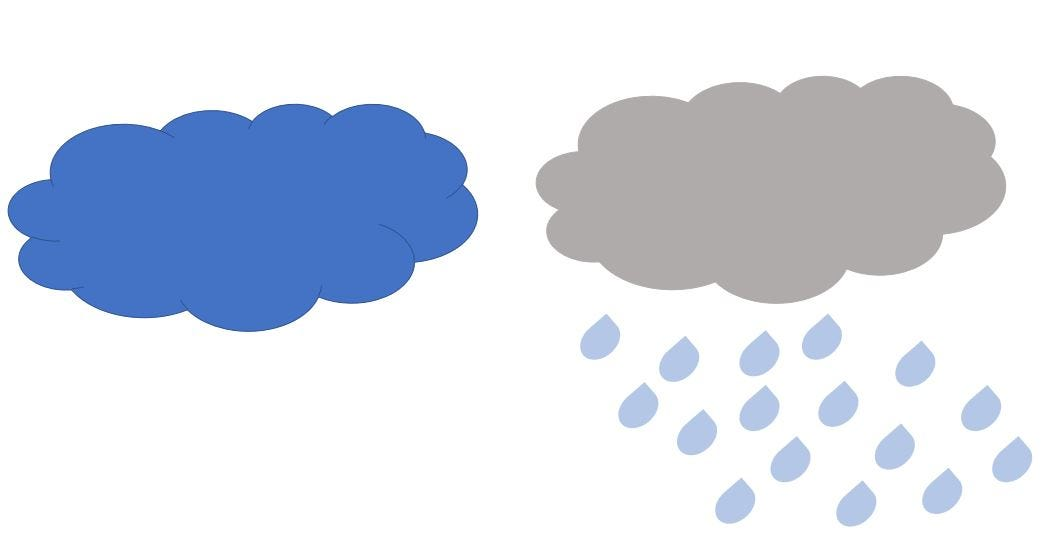# K Nearest Neighbors (K-NN) with numpy

Original article was published by Akshit Kothari on Artificial Intelligence on MediumStep 1: Import the libraries

`import pandas as pdimport numpy as npfrom collections import Counterfrom sklearn.model_selection import train_test_splitfrom sklearn.metrics import classification_report, accuracy_score`

Step 2: Read the data and pre-processing

`#Read the csv in the form of a dataframedf= pd.read_csv("data.csv")df.head()`
`#Removing the null valuesdf.dropna(axis=0, inplace=True)#Reset the index to avoid errordf.reset_index(drop=True, inplace=True)y = df['RAIN'].replace([False,True],[0,1])#Removing Date feature and Rain because it is our labeldf.drop(['RAIN','DATE'],axis=1,inplace=True) `
`#Splitting the data to train(75%) and test(25%)x_train,x_test,y_train,y_test=train_test_split(df,y,test_size=0.25)`

Step 3: Implementing Euclidean distance to find the nearest neighbor

Let’s try to understand Fig. 5 first, we have three features in two clusters, similar to our Seattle Rainfall data. Lets assume cluster 1 to be of data points when it did not rain and cluster 2 to be of data points when it did rain. The first two clusters can be thought of as our training data. The three data point outside the two clusters are the testing data for which we have to find the label.

Now, we will use Euclidean Distance to calculate the distance between the training data and the testing data. We can also use different methods to calculate distance like Manhatten Distance, Minkowski Distance etc.

Euclidean Distance:- It is used to find the straight line distance between two points.

Since, KNN is non-parametric i.e. it does not make any assumptions about the probability distribution of the input, we find the distance of test data points from the training data and the data points which are near to the test data are considered for classifying our test data. Let’s see this with the help of an image:-

We see that in the image we have 5 data points per feature, 2 classified in one cluster, 2 classified in other cluster and 1 not yet labelled. Once we calculate the euclidean distance between these data points we can classify the cluster where this would lie.

The K in KNN signifies the count which we have to consider, lets say K is provided 5, in this case it will consider top 5 shortest distances after which the class with more frequency will be considered as the class of the test data.

To understand the purpose of K we have taken only one independent variable as shown in Fig. 7 with 2 labels, i.e binary classification and after calculating the distances we have marked 5 nearest neighbors as K is assigned a value of 5. Now as we can see the frequency (3 for blue and 2 for red) of label in color blue is higher than for color red, we will label the test data point as blue. Finding out the value of K is a task in K-NN as it may require multiple iterations by trying different values and evaluating the model based on those values.

`def KNN(x,y,k):    dist = []     #Computing Euclidean distance    dist_ind = np.sqrt(np.sum((x-y)**2, axis=1))     #Concatinating the label with the distance    main_arr = np.column_stack((train_label,dist_ind))    #Sorting the distance in ascending order    main = main_arr[main_arr[:,1].argsort()]     #Calculating the frequency of the labels based on value of K    count = Counter(main[0:k,0])    keys, vals = list(count.keys()), list(count.values())    if len(vals)>1:        if vals>vals:            return int(keys)        else:            return int(keys)    else:        return int(keys)`

Step 4: Calculating the accuracy and classification report

`print(classification_report(pred,train_label))`
`print(classification_report(pred,test_label))`

Well we have achieved 96% in training data and 94% in test data, not bad!